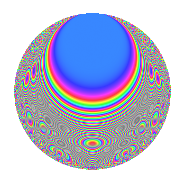# Properties

 Label 804.2.q.aLevel 804 Weight 2 Character orbit 804.q Analytic conductor 6.420 Analytic rank 0 Dimension 60 CM No

# Related objects

## Newspace parameters

 Level: $$N$$ = $$804 = 2^{2} \cdot 3 \cdot 67$$ Weight: $$k$$ = $$2$$ Character orbit: $$[\chi]$$ = 804.q (of order $$11$$ and degree $$10$$)

## Newform invariants

 Self dual: No Analytic conductor: $$6.41997232251$$ Analytic rank: $$0$$ Dimension: $$60$$ Relative dimension: $$6$$ over $$\Q(\zeta_{11})$$ Sato-Tate group: $\mathrm{SU}(2)[C_{11}]$

## $q$-expansion

The dimension is sufficiently large that we do not compute an algebraic $$q$$-expansion, but we have computed the trace expansion.

 $$\operatorname{Tr}(f)(q) =$$ $$60q - 6q^{3} - 2q^{5} - 2q^{7} - 6q^{9} + O(q^{10})$$ $$\operatorname{Tr}(f)(q) =$$ $$60q - 6q^{3} - 2q^{5} - 2q^{7} - 6q^{9} + 7q^{11} - 2q^{13} + 9q^{15} - 19q^{17} + 2q^{19} - 2q^{21} + 4q^{23} + 16q^{25} - 6q^{27} + 16q^{29} - 28q^{31} - 4q^{33} + 28q^{35} + 2q^{37} - 2q^{39} + 32q^{41} + 19q^{43} - 2q^{45} + 2q^{47} - 70q^{49} - 19q^{51} + 31q^{53} - 5q^{55} + 13q^{57} + 59q^{59} + 32q^{61} + 9q^{63} + 28q^{65} + 7q^{67} + 4q^{69} + 16q^{71} + 19q^{73} + 16q^{75} - 46q^{77} + 48q^{79} - 6q^{81} + 60q^{83} - 66q^{85} + 5q^{87} - 22q^{89} + 24q^{91} + 5q^{93} + 103q^{95} - 46q^{97} - 4q^{99} + O(q^{100})$$

## Embeddings

For each embedding $$\iota_m$$ of the coefficient field, the values $$\iota_m(a_n)$$ are shown below.

For more information on an embedded modular form you can click on its label.

Label $$a_{2}$$ $$a_{3}$$ $$a_{4}$$ $$a_{5}$$ $$a_{6}$$ $$a_{7}$$ $$a_{8}$$ $$a_{9}$$ $$a_{10}$$
25.1 0 −0.654861 + 0.755750i 0 −2.85861 + 1.83711i 0 −0.661793 4.60287i 0 −0.142315 0.989821i 0
25.2 0 −0.654861 + 0.755750i 0 −2.63012 + 1.69027i 0 0.473818 + 3.29548i 0 −0.142315 0.989821i 0
25.3 0 −0.654861 + 0.755750i 0 0.361898 0.232578i 0 −0.272618 1.89610i 0 −0.142315 0.989821i 0
25.4 0 −0.654861 + 0.755750i 0 0.412663 0.265202i 0 0.411742 + 2.86373i 0 −0.142315 0.989821i 0
25.5 0 −0.654861 + 0.755750i 0 1.20911 0.777049i 0 −0.250069 1.73927i 0 −0.142315 0.989821i 0
25.6 0 −0.654861 + 0.755750i 0 3.26560 2.09868i 0 0.572019 + 3.97848i 0 −0.142315 0.989821i 0
193.1 0 −0.654861 0.755750i 0 −2.85861 1.83711i 0 −0.661793 + 4.60287i 0 −0.142315 + 0.989821i 0
193.2 0 −0.654861 0.755750i 0 −2.63012 1.69027i 0 0.473818 3.29548i 0 −0.142315 + 0.989821i 0
193.3 0 −0.654861 0.755750i 0 0.361898 + 0.232578i 0 −0.272618 + 1.89610i 0 −0.142315 + 0.989821i 0
193.4 0 −0.654861 0.755750i 0 0.412663 + 0.265202i 0 0.411742 2.86373i 0 −0.142315 + 0.989821i 0
193.5 0 −0.654861 0.755750i 0 1.20911 + 0.777049i 0 −0.250069 + 1.73927i 0 −0.142315 + 0.989821i 0
193.6 0 −0.654861 0.755750i 0 3.26560 + 2.09868i 0 0.572019 3.97848i 0 −0.142315 + 0.989821i 0
241.1 0 0.415415 0.909632i 0 −3.88811 1.14165i 0 1.58324 + 1.82715i 0 −0.654861 0.755750i 0
241.2 0 0.415415 0.909632i 0 −1.26159 0.370436i 0 −2.70108 3.11721i 0 −0.654861 0.755750i 0
241.3 0 0.415415 0.909632i 0 −0.925675 0.271803i 0 1.06841 + 1.23301i 0 −0.654861 0.755750i 0
241.4 0 0.415415 0.909632i 0 0.346311 + 0.101686i 0 −0.316787 0.365591i 0 −0.654861 0.755750i 0
241.5 0 0.415415 0.909632i 0 2.96527 + 0.870683i 0 2.08291 + 2.40380i 0 −0.654861 0.755750i 0
241.6 0 0.415415 0.909632i 0 4.02046 + 1.18051i 0 −1.53030 1.76606i 0 −0.654861 0.755750i 0
265.1 0 −0.142315 + 0.989821i 0 −1.58453 3.46964i 0 −2.06192 0.605433i 0 −0.959493 0.281733i 0
265.2 0 −0.142315 + 0.989821i 0 −1.15584 2.53094i 0 2.78570 + 0.817955i 0 −0.959493 0.281733i 0
See all 60 embeddings
 $$n$$: e.g. 2-40 or 990-1000 Embeddings: e.g. 1-3 or 685.6 Significant digits: Format: Complex embeddings Normalized embeddings Satake parameters Satake angles

## Inner twists

This newform does not have CM; other inner twists have not been computed.

## Hecke kernels

This newform can be constructed as the kernel of the linear operator $$T_{5}^{60} + \cdots$$ acting on $$S_{2}^{\mathrm{new}}(804, [\chi])$$.